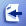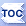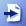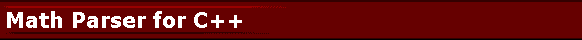bcParserCPP is a formula parser class for C++. It makes it possible for your application to parse and evaluate a mathematical expression string at runtime.

The Math Parser for C++ product comes as a CMathParser C++ Template Class which you can easily include into your C++ projects and parse mathematical expressions with no effort.

This C++ template resides in MathParser.h and it takes template parameters to define which numeric and character types to use. It is customary to use the parser with double / long double precision. However, another C++ type that implements necessary operators could be used too. The pluggable data type parameter opens the door for mathematical operations on candidates like Complex numbers, Rational numbers, and Arbitrary Precision types that perform arbitrary precision arithmetic.

 A Happy User Says: I purchased the parser yesterday and it dropped right into the project with minimal effort. It seems to be working perfectly. Thanks for your replies to my queries!--Tom ` `
MathParser.h is delivered as standard C++ source code ready to include in any C++ project with 1 line. MathParser.h can be used with Visual C++ (Any version), GNU C++ Compiler (3.x and above) and possibly others.

Here is an example command line expression Calculator developed using CMathParser template class. Here is Math Parser for C++ Documentation generated by Doxygen (Many thanks to Doxygen).

Example of using the math parser with MAPM, a decimal math library for C++ for greater precision arithmetic.

Download demo Calculator.exe command line math expression calculator for Windows (It can be compiled for Linux and Mac OS as well as Windows).

# Mathematical Expression Parser for C++

bcParserCPP Math Parser for C++ library features include:

• Easy to use, simple C++ API.
• Comes with predefined functions.
• You can create custom functions/variables and get a callback to your functions that you define in your source code.
• VariableCallback to provide values for undefined variables.
• Optimization: Constant expression elimination for repeated tasks.
• Operators: +, -, /, *, ^
• Logical Operators: <, >, =, <>, >=, <=, &, |, ! [IF(condition,case1,case2) is supported]
• Paranthesis: (, {, [
• Functions in the form of: f(x1, x2, ..., xN)
• Common math.h functions predefined.
• Pluggable numeric and character types via C++ Template mechanism.
• Royalty free distribution for your binaries.
• Portable (Windows, Linux, Mac OSX) C++ Source code is included.
• bcParserCPP, Math Parser for C++, is especially useful in scientific, engineering programs as well as financial spread sheet implementations.

Sample screen shots of Math Parser use in a C++ application in Eclipse:

Here is C++ Console Application source code using bcParserCPP, Math Parser for C++:

 #include #define _DOUBLE #include "MathParser.h" using namespace std; //define a Math Parser that works with char strings for //variable and function names, and double values. typedef CMathParser MathParser; /**  * User defined function that multiplies all parameters with each other and returns the result.  * Can be used with a math parser that works with 'double' values.  * (Hint: Use C++ templates to create functions that work with parametrized types)  */ double mult(MathParser* pParentParser, const double p[], const int count){     double result = 1;     for(int i=0; i." << endl;         }catch(...){                 cout << "Unexpected error in math parser." << endl;         } }

ParserException::GetInvalidPortionOfExpression function reports invalid section of the expression that caused ParserException. This can be used to conclude whether you need to define a variable on the fly or not. Useful in cases where the names of variables are not known ahead of time. You can then define the variable and try again.

Choosing the desired numeric type by using a #define before you include MathParser.h:

//If you are going to use the parser with std::complex, then define this.
#define _COMPLEX

//If you are going to use the parser with float, double, long double, then define this.
#define _DOUBLE

//If you are going to use the parser with a arbitrary precision decimal type such as MAPM then define this.
#define _DECIMAL

These defines affect what and how default functions and variables are implemented and defined. Other numeric types that are not covered by default may require changes to the default functions as well.

The Math Parser Component is also available on many platforms/languages such as:

Examples of typical expressions are:

 SIN(3.14)+5^2+POW(2,MAX(X* 2,Y)) Functions, variables, constants can be nested.Common math functions are defined by default. 2*[LN(1+X) / LOG(1-X)] Paranthesis can be (, {, [ for readability. IF(X>0, 3/X, F(X))SUM(X,Y,3,4) IF(a,b,c) branching function is supported. Boolean operators are supported. Any non-zero value is TRUE, 0 is FALSE. Functions can be defined to have any number of parameters. PI*(R^2) Constants supported. For example, PI is a constant, not a variable. When optimization is turned on, constants may improve the speed of repeated evaluations where expression does not change but variable values change. X+Y/LOG(1+5) If Optimization is turned ON, LOG(1+5) will be optimized away since it is a constant. VOLUME(HEIGHT, WIDTH, LENGTH) You can create your own functions and variables and name them as you wish. You can replace a predefined function with your own implementation. X+COSH(3E-2) Scientific notation is supported: 3E-2

To be efficient in repeated calculations, parser creates a parse tree at first and reuses this parse tree for each evaluation without the need to reparse.

Optimizer: If Optimization is on, the parse tree will be optimized by calculating constant expression sections at once so that further evaluation requests will be quicker without the need to re-evaluate those constant branches.

bcParserCPP, the Math Parser for C++, can typically be used with Visual C++, C++ Builder, GNU C++ and other C++ compilers that can handle C++ templates. We have tested it with Visual C++ and GNU C++ compilers.

Parser is delivered as a zip file that contains the MathParser.h and it comes with a help file for reference and a C++ demo application for aid in getting started.

Zip file contains the the MathParser.h,  license agreement, a help file and a demo C++ MathParserTest.cpp source file.

Here is an example command line expression Calculator developed using CMathParser template class.